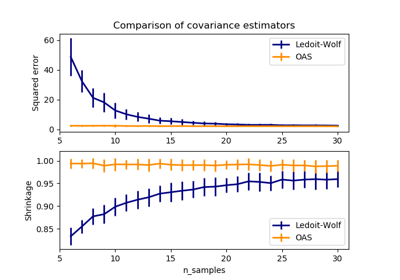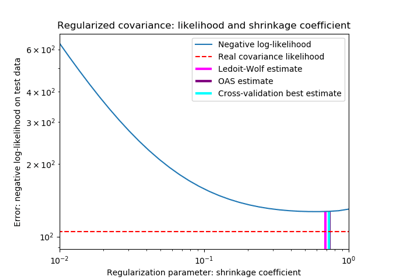/scikit-learn

# sklearn.covariance.OAS

`class sklearn.covariance.OAS(store_precision=True, assume_centered=False)` [source]

Oracle Approximating Shrinkage Estimator

Read more in the User Guide.

OAS is a particular form of shrinkage described in “Shrinkage Algorithms for MMSE Covariance Estimation” Chen et al., IEEE Trans. on Sign. Proc., Volume 58, Issue 10, October 2010.

The formula used here does not correspond to the one given in the article. In the original article, formula (23) states that 2/p is multiplied by Trace(cov*cov) in both the numerator and denominator, but this operation is omitted because for a large p, the value of 2/p is so small that it doesn’t affect the value of the estimator.

Parameters: `store_precision : bool, default=True` Specify if the estimated precision is stored. `assume_centered : bool, default=False` If True, data are not centered before computation. Useful when working with data whose mean is almost, but not exactly zero. If False (default), data are centered before computation. `covariance_ : array-like, shape (n_features, n_features)` Estimated covariance matrix. `precision_ : array-like, shape (n_features, n_features)` Estimated pseudo inverse matrix. (stored only if store_precision is True) `shrinkage_ : float, 0 <= shrinkage <= 1` coefficient in the convex combination used for the computation of the shrunk estimate.

#### Notes

The regularised covariance is:

(1 - shrinkage) * cov + shrinkage * mu * np.identity(n_features)

where mu = trace(cov) / n_features and shrinkage is given by the OAS formula (see References)

#### References

“Shrinkage Algorithms for MMSE Covariance Estimation” Chen et al., IEEE Trans. on Sign. Proc., Volume 58, Issue 10, October 2010.

#### Methods

 `error_norm`(comp_cov[, norm, scaling, squared]) Computes the Mean Squared Error between two covariance estimators. `fit`(X[, y]) Fits the Oracle Approximating Shrinkage covariance model according to the given training data and parameters. `get_params`([deep]) Get parameters for this estimator. `get_precision`() Getter for the precision matrix. `mahalanobis`(X) Computes the squared Mahalanobis distances of given observations. `score`(X_test[, y]) Computes the log-likelihood of a Gaussian data set with `self.covariance_` as an estimator of its covariance matrix. `set_params`(**params) Set the parameters of this estimator.
`__init__(store_precision=True, assume_centered=False)` [source]
`error_norm(comp_cov, norm=’frobenius’, scaling=True, squared=True)` [source]

Computes the Mean Squared Error between two covariance estimators. (In the sense of the Frobenius norm).

Parameters: `comp_cov : array-like, shape = [n_features, n_features]` The covariance to compare with. `norm : str` The type of norm used to compute the error. Available error types: - ‘frobenius’ (default): sqrt(tr(A^t.A)) - ‘spectral’: sqrt(max(eigenvalues(A^t.A)) where A is the error `(comp_cov - self.covariance_)`. `scaling : bool` If True (default), the squared error norm is divided by n_features. If False, the squared error norm is not rescaled. `squared : bool` Whether to compute the squared error norm or the error norm. If True (default), the squared error norm is returned. If False, the error norm is returned. The Mean Squared Error (in the sense of the Frobenius norm) between `self` and `comp_cov` covariance estimators.
`fit(X, y=None)` [source]

Fits the Oracle Approximating Shrinkage covariance model according to the given training data and parameters.

Parameters: `X : array-like, shape = [n_samples, n_features]` Training data, where n_samples is the number of samples and n_features is the number of features. y not used, present for API consistence purpose. `self : object`
`get_params(deep=True)` [source]

Get parameters for this estimator.

Parameters: `deep : boolean, optional` If True, will return the parameters for this estimator and contained subobjects that are estimators. `params : mapping of string to any` Parameter names mapped to their values.
`get_precision()` [source]

Getter for the precision matrix.

Returns: `precision_ : array-like` The precision matrix associated to the current covariance object.
`mahalanobis(X)` [source]

Computes the squared Mahalanobis distances of given observations.

Parameters: `X : array-like, shape = [n_samples, n_features]` The observations, the Mahalanobis distances of the which we compute. Observations are assumed to be drawn from the same distribution than the data used in fit. `dist : array, shape = [n_samples,]` Squared Mahalanobis distances of the observations.
`score(X_test, y=None)` [source]

Computes the log-likelihood of a Gaussian data set with `self.covariance_` as an estimator of its covariance matrix.

Parameters: `X_test : array-like, shape = [n_samples, n_features]` Test data of which we compute the likelihood, where n_samples is the number of samples and n_features is the number of features. X_test is assumed to be drawn from the same distribution than the data used in fit (including centering). y not used, present for API consistence purpose. `res : float` The likelihood of the data set with `self.covariance_` as an estimator of its covariance matrix.
`set_params(**params)` [source]

Set the parameters of this estimator.

The method works on simple estimators as well as on nested objects (such as pipelines). The latter have parameters of the form `<component>__<parameter>` so that it’s possible to update each component of a nested object.

Returns: self

## Examples using `sklearn.covariance.OAS`Ledoit-Wolf vs OAS estimationShrinkage covariance estimation: LedoitWolf vs OAS and max-likelihood